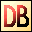DelphiBasics
 SinFunction The Sine of a number System unit
 function Sin(const Number Extended):Extended;
Description
The Sin function is a mathematical function giving the Sine value of a Number value given radians.

Notes
The System unit supports only the following angular functions:

ArcTan
Sin
Cos

Related commands
 ArcCos The Arc Cosine of a number, returned in radians ArcSin The Arc Sine of a number, returned in radians ArcTan The Arc Tangent of a number, returned in radians Cos The Cosine of a number Tan The Tangent of a numberDownload this web site as a Windows program.

 Example code : Get the Sine of 30 degrees var   float : single; begin   // The Sine of 30 degrees = 0.5   float := Sin(PI/6);  // = 180/6 = 30 degrees   ShowMessage('Sin(PI/6) = '+FloatToStr(float)); end; Show full unit code Sin(PI/6) = 0.5